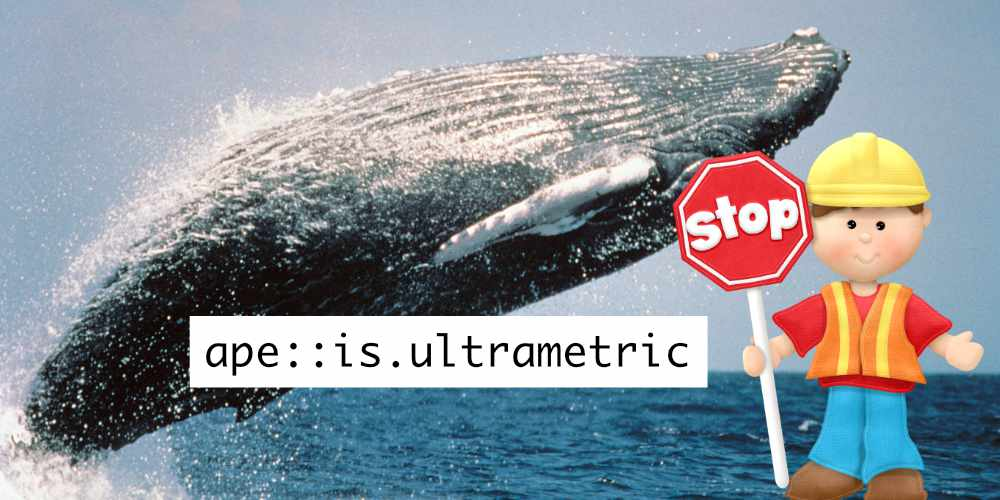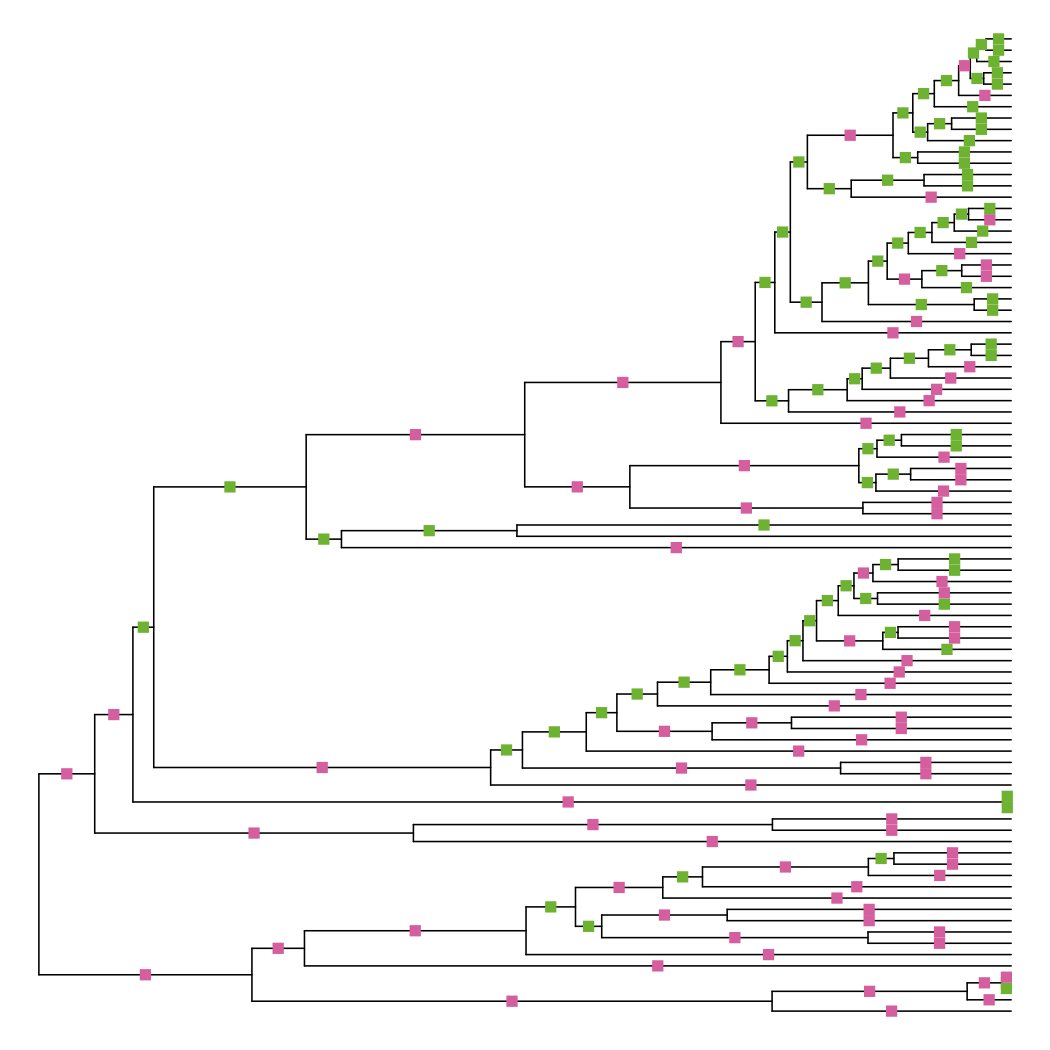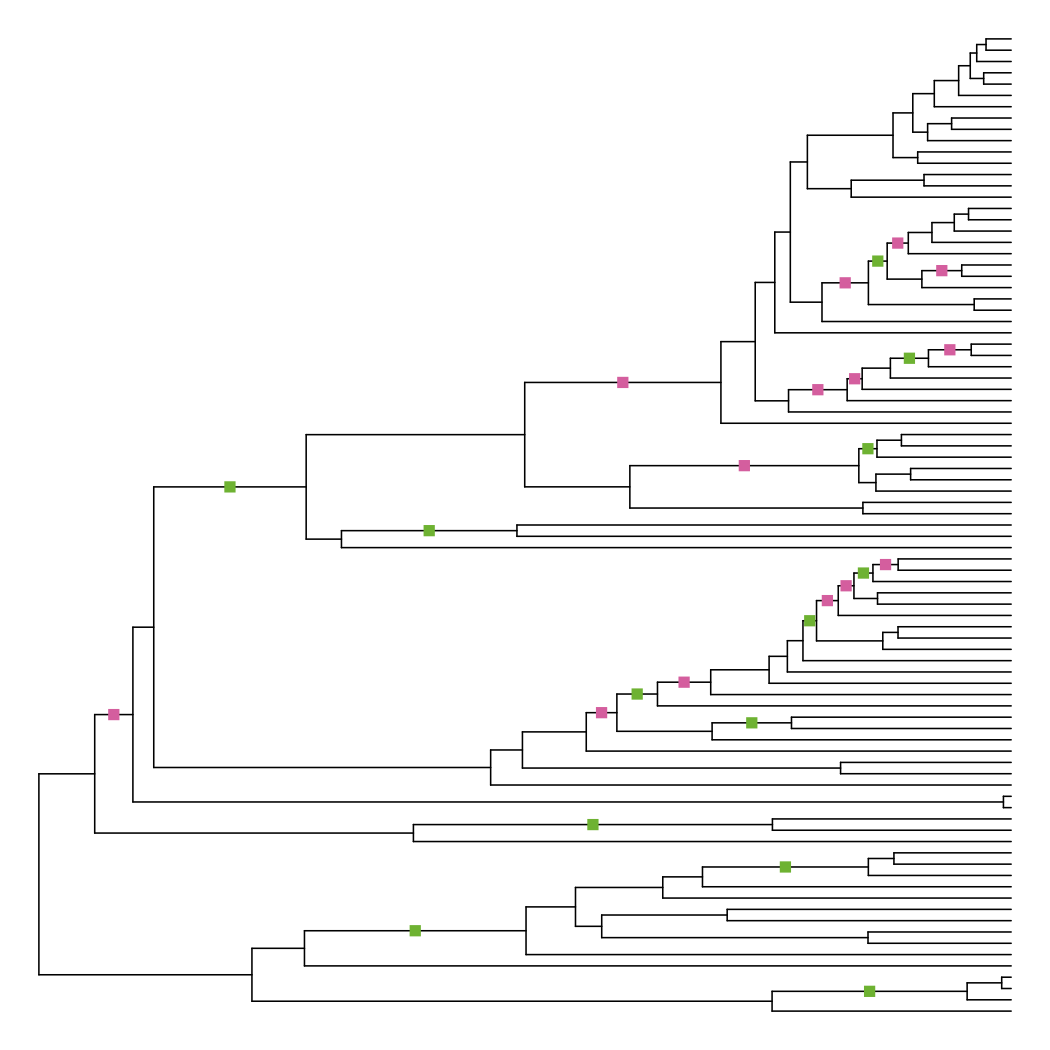# Three ways to check and fix ultrametric phylogenies

A recent user bug report in my software TACT led me to look into how phylogenetic software varies in the way they determine whether a given phylogenetic tree is ultrametric (where the root-to-tip distance is equal among all tips). If you infer an ultrametric phylogeny using something like BEAST or treePL, your supposedly ultrametric tree can still cause problems for other tools by virtue of not being ultrametric enough.

This ultrametric purity test was previously a problem during the great BAMM controversy of 2017 (“BAMMghazi”), when the whales phylogeny used as an example in BAMM suddenly stopped being ultrametric as measured by the R function `ape::is.ultrametric`.

How then, do tools differ in the way that they check for ultrametricity?## Method 1: variance

This is used in phyx, and was used in ape prior to version 4.0.

Load the whales tree into R and compute the root-to-tip distances for all tips:

``````tre <- read.tree("https://raw.githubusercontent.com/macroevolution/bamm/ab1b69be13e9841d9e103170d0f61e4324f78676/examples/diversification/whales/whaletree.tre")

N <- Ntip(tre)
root_node <- N + 1
root_to_tip <- dist.nodes(tre)[1:N, root_node]
``````

Compute the variance using those distances:

``````var(root_to_tip)
##  6.519647e-13
``````

The default tolerance in ape is the square root of R’s machine epsilon, defined as the smallest positive floating-point number x such that 1 + x ≠ 1. This can vary from computer to computer, but on my laptop, this value is:

``````sqrt(.Machine\$double.eps)
##  1.490116e-08
``````

The whales tree is ultrametric using the variance method, since 1e-8 is much larger than 1e-13.

## Method 2: relative difference

In ape 4.0, the `is.ultrametric` method was changed to use the relative difference of the minimum and maximum root-to-tip distances. I’ll get into why this was changed, but first, let’s look at how to calculate this value:

``````min_tip <- min(root_to_tip)
max_tip <- max(root_to_tip)
(max_tip - min_tip) / max_tip
##  1.115516e-07
``````

Immediately we can see why the whales tree stopped being ultrametric in ape 4.0, as this relative difference is larger than the default tolerance.

The default criterion is invariant to linear changes of the branch lengths.

What does this look like in practice? Let’s first scale the root-to-tip distance by multiplying everything by 1000:

``````scaled_root_to_tip <- root_to_tip * 1000
``````

Now compare the variance and relative difference:

``````var(scaled_root_to_tip)
##  6.519647e-07

min_tip <- min(scaled_root_to_tip)
max_tip <- max(scaled_root_to_tip)
(max_tip - min_tip) / max_tip
##  1.115516e-07
``````

Uh oh! Our whales are no longer ultrametric per the variance statistic, even though none of the branch lengths have changed relative to each other. You may not think that your phylogenies should be stretching like a taffy pull, but there are probably valid use cases for phylogenies like this.

This is the method that TACT currently uses to determine ultrametricity.

## Method 3: node ages

The previous two methods all relied on comparing some aspect of root-to-tip distances. Here, we’ll actually compare the distances to the tips of all nodes. This is the method used in DendroPy and the original impetus for this investigation. I’ll reimplement enough of this method in R to illustrate this technique.

First, reorder the tree for postorder traversal, and set up some convenience variables.

``````tre_node_adjust <- reorder(tre, "postorder")

e1 <- tre_node_adjust\$edge[, 1] # parent node
e2 <- tre_node_adjust\$edge[, 2] # child node
``````

Also set up an `ages` variable that will hold internal calculations for how old a node should be.

``````ages <- numeric(N + tre_node_adjust\$Nnode)
``````

Next, start iterating…

``````for (ii in seq_along(EL)) {
``````

If we haven’t already stored an age for the parent node, go ahead and compute that now from the (left)1 child node and the current edge length.

``````    if (ages[e1[ii]] == 0) {
ages[e1[ii]] <- ages[e2[ii]] + EL[ii]
``````

Otherwise, retrieve the stored age for the parent node, and re-compute what the age should be based on the (right)1 child node.

``````    } else {
recorded_age <- ages[e1[ii]]
new_age <- ages[e2[ii]] + EL[ii]
``````

Now test whether those ages differ. I could actually use either the variance or the relative difference method, but here I’ll just check for absolute difference.

``````        if (recorded_age != new_age) {
cat(sprintf("node %i age %.6f != %.6f\n", e1[ii], recorded_age, new_age))
}
}
}

## node 154 age 3.291163 != 3.291164
## node 153 age 4.570892 != 4.570893
## node 151 age 5.263495 != 5.263494
## node 150 age 6.975185 != 6.975185
## node 146 age 3.048675 != 3.048675
## node 145 age 4.452720 != 4.452720
## node 143 age 6.047030 != 6.047031
## node 142 age 8.209050 != 8.209050
## node 135 age 5.616381 != 5.616380
## node 133 age 14.061554 != 14.061555
## node 132 age 17.939426 != 17.939427
## node 130 age 24.698214 != 24.698213
## node 127 age 5.096384 != 5.096384
## node 125 age 5.796587 != 5.796586
## node 124 age 6.375631 != 6.375632
## node 121 age 7.176680 != 7.176680
## node 120 age 7.677424 != 7.677424
## node 116 age 13.042869 != 13.042870
## node 114 age 11.028304 != 11.028304
## node 113 age 14.540283 != 14.540283
## node 112 age 15.669702 != 15.669702
## node 108 age 31.621529 != 31.621528
## node 104 age 22.044391 != 22.044391
## node 103 age 33.799003 != 33.799004
## node 100 age 11.382958 != 11.382958
## node 93 age 26.063016 != 26.063016
## node 90 age 8.816019 != 8.816019
``````

Many internal nodes have ages that differ depending on whether you use the left or right child node to compute the age. This metric allows you to pinpoint exactly where in your phylogeny precision issues could be causing ultrametricity issues. I can imagine this being quite useful when you are grafting subtrees onto a backbone phylogeny and trying to figure out if you did the math on your branch lengths correctly.

## Fix 1: extending the tips

Now that we’re aware of the differences between different ultrametricity checks, let’s look at ways to correct for phylogenies that aren’t quite there.

One possibility is to simply extend the tips of the tree until the root-to-tip distances are completely equal. This is implemented in R as `BioGeoBEARS::extend_tips_to_ultrametricize`2 and `phytools::force.ultrametric(method = "extend")`.3

First, set up a copy of the whales tree and compute some important values, including the difference from each root-to-tip distance to their maximum:

``````tre_extend <- tre
age_difference <- max(root_to_tip) - root_to_tip
``````

Next, grab the edges from the edge matrix that correspond to the tips. Note that the edges in `\$edge.label` correspond to the row numbers in `\$edge`, not the values in those rows! This is tricky and confusing. We can also assume that the tip edges appear in ascending order, from 1 to N.

``````tip_edges <- tre_extend\$edge[, 2] <= Ntip(tre_extend)
``````

Finally, extend the tips outwards and confirm that the new tree is ultrametric:

``````tre_extend\$edge.length[tip_edges] <- tre_extend\$edge.length[tip_edges] + age_difference
is.ultrametric(tre_extend)
##  TRUE
``````

We can write a simple function in R that compares two phylogenies with identical topologies but differing branch lengths.

``````diff_edge_lengths <- function(phy, phy2) {
diffs <- phy2\$edge.length - phy\$edge.length
``````

Use the `sign` function, which returns -1, 0, or 1 when the input is negative, zero, or positive, respectively. Then assign each of those values to a color (or lack thereof). This is ColorBrewer palette PiYG.

``````    cols <- sign(diffs)
cols[cols == 1] <- "#7fbc41"
cols[cols == -1] <- "#de77ae"
cols[cols == 0] <- NA
``````

Plot the tree and report the results. The plot needs a bit of adjustment since the defaults of `ape::plot.phylo` are a bit aggravating.

``````    plot(phy, show.tip.label = FALSE, no.margin = TRUE)
edgelabels(pch = 15, col = cols)
sprintf("%i longer branches, %i shorter branches", sum(diffs > 0), sum(diffs < 0))
}
``````

This function places squares on branches that have changed in length, with red meaning a shorter branch and green meaning a longer branch. As expected, nearly all of the terminal branches have been extended to enforce ultrametricity.

``````diff_edge_lengths(tre, tre_extend)
##  "85 longer branches, 0 shorter branches"
``````## Fix 2: non-negative least squares

This is the default approach used in the R function `phytools::force.ultrametric` and the topic of a phytools blog post.

This will give you the edge lengths that result in the distances between taxa with minimum sum of squared differences from the distances implied by your input tree, under the criterion that the resulting tree is ultrametric.

``````tre_nnls <- phangorn::nnls.tree(cophenetic(tre), tre, rooted = TRUE)
is.ultrametric(tre_nnls)
##  TRUE
``````

Since it’s trying to minimize differences among the pairwise tip distance matrix, you’d expect many branches to be adjusted. Plotting the differences show that this is indeed the case:

``````diff_edge_lengths(tre, tre_nnls)
##  "86 longer branches, 85 shorter branches"
``````This is the approach optionally used in DendroPy,4 and is how TACT fixes ultrametricity issues if asked. Whenever a node’s age differs between its left and right children, correct one of the branch lengths so the node’s age will be calculated the same regardless of whether you’re using the left descendants or the right descendants.1

Change the prior loop that checks node ages to additionally adjust the branch length:

`````` for (ii in seq_along(EL)) {
if (ages[e1[ii]] == 0) {
ages[e1[ii]] <- ages[e2[ii]] + EL[ii]
} else {
recorded_age <- ages[e1[ii]]
new_age <- ages[e2[ii]] + EL[ii]
if (recorded_age != new_age) {
cat(sprintf("node %i age %.6f != %.6f\n", e1[ii], recorded_age, new_age))
+            EL[ii] <- recorded_age - ages[e2[ii]]
}
}
}
``````

Then, update the branch lengths in the phylogeny itself and confirm that it’s ultrametric.

``````tre_node_adjust\$edge.length <- EL
##  TRUE
``````

Plotting the differences shows that this method actually changes the fewest number of branches.

``````diff_edge_lengths(tre, tre_node_adjust)
##  "13 longer branches, 14 shorter branches"
``````## Issues with large phylogenies

Another issue identified in the bug report was the inability to use `phytools::force.ultrametric` on large phylogenies. This is indeed the case when testing a random tree with 50,000 tips.

``````library(ape)
(xx <- rcoal(50000))
## Phylogenetic tree with 50000 tips and 49999 internal nodes.
##
## Tip labels:
##   t11339, t29898, t18919, t6336, t34524, t1665, ...
##
## Rooted; includes branch lengths.

is.ultrametric(xx)
##  TRUE

phytools::force.ultrametric(xx, method = "nnls")
## Error in double(nm * nm) : vector size cannot be NA
## In nm * nm : NAs produced by integer overflow

phytools::force.ultrametric(xx, method = "extend")
## Error: vector memory exhausted (limit reached?)
``````

With `method = "extend"`, its current implementation calls `diag(vcv(tree))`, which requires creating an N by N matrix as a temporary value, where N is the number of tips. With 50,000 tips this implies a vector of length 2.5 billion, which exceeds R’s vector limit of 2.1 billion. This function could be optimized to avoid this storage requirement. With `method = "nnls"` creating this N by N matrix may be unavoidable, so for large phylogenies consider using the tip extension or the node adjustment methods instead.

## Closing thoughts

None of this really matters anyway, except in the special case of really big phylogenies, and even then not so much. If you’re certain your phylogeny is ultrametric, use any of these methods and you’ll be able to get your tree to be so precisely ultrametric that even the strictest tools can’t complain.

I’m serious! Look at how well the “extend tips” method works:

``````is.ultrametric(tre_extend, tolerance = 0)
##  TRUE
``````

This means there are literally no differences in root-to-tip distances even to a precision of 16 digits. This is enough to leave the solar system, so it’s probably good enough for your phylogeny, which likely has more interesting sources of error beyond numerical precision.

## Notes

1. Left and right are arbitrary here and named only to help the reader distinguish between the two.

2. BioGeoBEARS also has a function to average the heights of tip nodes.

3. You can also read my embarrassing, non-working original attempt. I’ve gotten better at R, I promise.

4. Note that DendroPy lets you pick either the maximum or the minimum implied ages; my implementation here just picks the first one it sees, so is sensitive to rearrangements such as from a ladderized tree.

If you found this post useful, please consider supporting my work with a local brew 🍺.